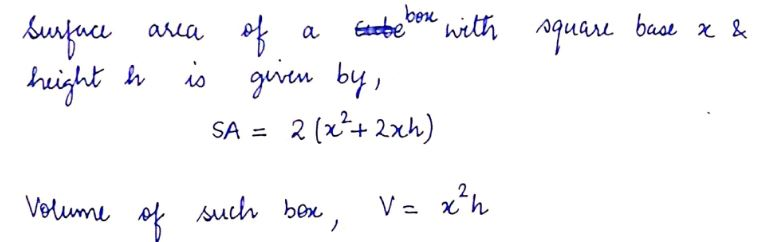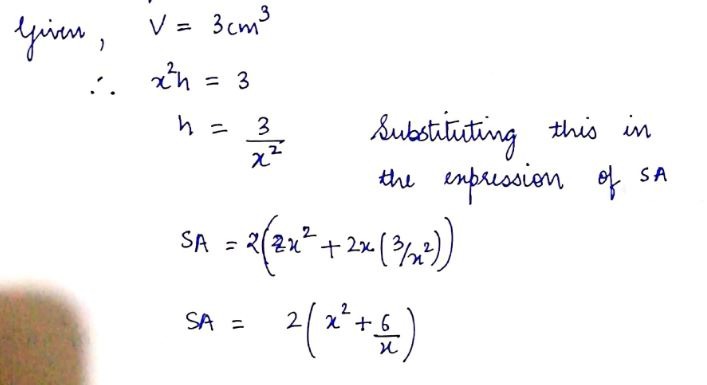# For a closed rectangular box, with a square base x by x cm and height h cm, find the dimensions giving the minimum surface area, given that the volume is 3 cm3. What are the exact answers for x and h?

Question
5 views

For a closed rectangular box, with a square base x by x cm and height h cm, find the dimensions giving the minimum surface area, given that the volume is 3 cm3. What are the exact answers for x and h?

check_circle

star
star
star
star
star
1 Rating
Step 1Step 2...

### Want to see the full answer?

See Solution

#### Want to see this answer and more?

Solutions are written by subject experts who are available 24/7. Questions are typically answered within 1 hour.*

See Solution
*Response times may vary by subject and question.
Tagged in